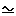# Maths - Fundamental Homomorphism Theorem

The fundamental homomorphism theorem states that. If φ: G->H is a homomorphism, then
Im(φ)G / Ker(φ)

### Example: D3 to C3

Lets look at a morphism from D3 to C3:So, in this case,

• Im(φ) = 1,r,r²
• G = {1,1}, {r,1}, {r²,1},{1,m}, {r,m}, {r²,m}
• Ker(φ) = {1,1},{1,m}

So if we divide {1,1}, {r,1}, {r²,1},{1,m}, {r,m}, {r²,m} by {1,1},{1,m} we get
{1,N}, {r,N}, {r²,N}
where N stands for the larger circles in the diagram.

We can see that the group of big circles is isomorphic to C3 and so Im(φ)G / Ker(φ) as required.

Now lets look at a morphism, in the other direction, from C3 to D3:So, in this case,

• Im(φ) = {1,1}, {r,1}, {r²,1}
• G = 1,r,r²
• Ker(φ) = e

So this is relatively trivial as it is saying that 1,r,r² is isomorphic to {1,1}, {r,1}, {r²,1}

### Futher Study

Where I can, I have put links to Amazon for books that are relevant to the subject, click on the appropriate country flag to get more details of the book or to buy it from them.Contemporary Abstract Algebra written by Joseph Gallian - This investigates algebra (Groups, Rings, Fields) using an abstract (set theory) approach. It is very readable for such an abstract topic but the inclusion of 1 page histories of the great mathematicians and other background information.